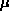### Simulation solutions of the Stochastic Differential Equation dW =dt +dX

This is the simplest stochastic differential equation -- its solution is a Wiener process (Brownian motion) with drift coefficientand diffusion coefficient.

By the analysis in the preceding lecture notes, we know to expect the solution W(t) to be a random variable, normally distributed with meant and standard deviationsqrt(t).

In this simulation, you get to use the slider bars to setand, and then have the simulation repeatedly construct a (Brownian) path with the parameters you have chosen. You can compare the stochastic simulation (blue graph) with the solution of the deterministic differential equation dW=dt (pink graph). At the right of the graph, the number of times the path ends in each of the intervals delineated by the black lines is tallied (after you setand, you can choose how many intervals you would like to consider).

After you have done a number of simulations (you may need to do at least one or two hundred to get good statistics) you can see the results of the simulation (for t=10) summarized against the theoretical probability distribution. In order to help you complete a large number of simulations quickly, you may click on the "many" button to do them 25 at a time.

#### Step-by-Step Instructions:

• Use slider bars to setand.
• Choose the number of W-axis subdivisions (4, 12, 20 or 40).
• Click on the "once" button to run the simulation once, or the "many" button for 25 runs at a time. Repeat this until you feel you have enough samples.
• Click "summarize" to compare your simulation to the theoretical probability distribution for t=10.
• Click "reset" (at any time) to start over.